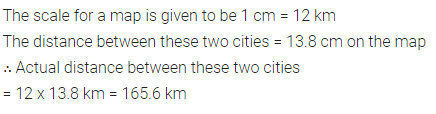# Selina Concise Mathematics Class 7 ICSE Solutions Chapter 18 Recognition of Solids

## Selina Concise Mathematics Class 7 ICSE Solutions Chapter 18 Recognition of Solids (Representing 3-D in 2-D)

Selina Publishers Concise Mathematics Class 7 ICSE Solutions Chapter 18 Recognition of Solids (Representing 3-D in 2-D)

### Recognition of solids Exercise 18 – Selina Concise Mathematics Class 7 ICSE Solutions

Question 1.
Identify the nets which can be used to form cubes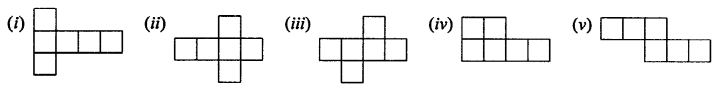Solution:Question 2.
Draw at least three different nets for making cube.
Solution: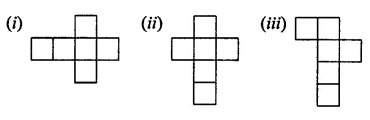Question 3.
The figure, given below, shows shadows of some 3D objects, when seen under the lamp of an overhead projector :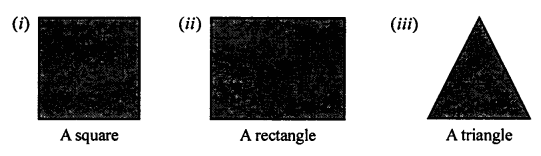In each case, name the object.
Solution:Question 4.
Using Euler’s formula, find the values of a, b, c and d.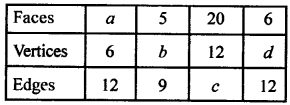Solution: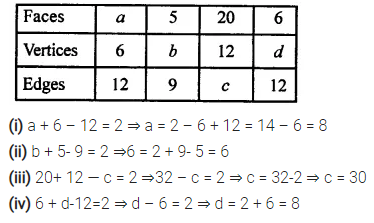Question 5.
Dice are cubes with dot or dots on each face. Opposite faces of a die always have a total of seven on them.
Below are given two nets to make dice (cube), the numbers inserted in each square indicate the number of dots in it.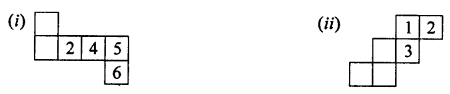Insert suitable numbers in each blank so that numbers in opposite faces of the die have a total of seven dots.
Solution:Question 6.
The following figures represent nets of some solids. Name the solids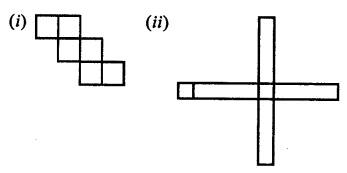Solution: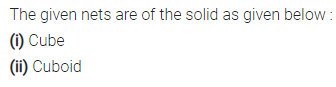Question 7.
Draw a map of your class room using proper scale and symbols for different objects.
Solution: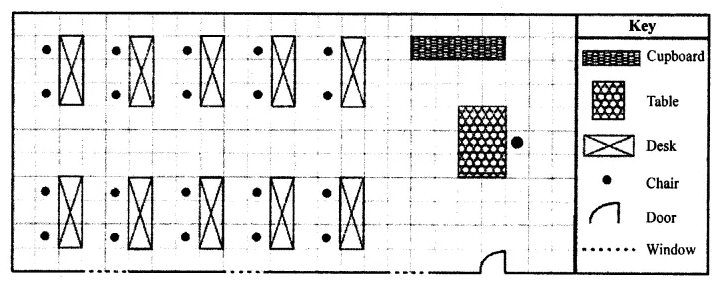Question 8.
Draw a map of your school compound using proper scale and symbols for various features like play ground, main building, garden, etc.
Solution:Question 9.
In the map of India, the distance between two cities is 13.8 cm.
Taking scale : 1 cm = 12 km, find the actual distance between these two cities.
Solution: